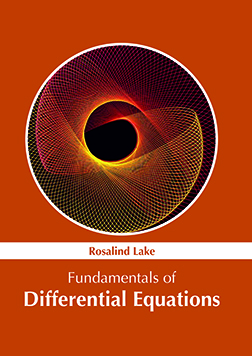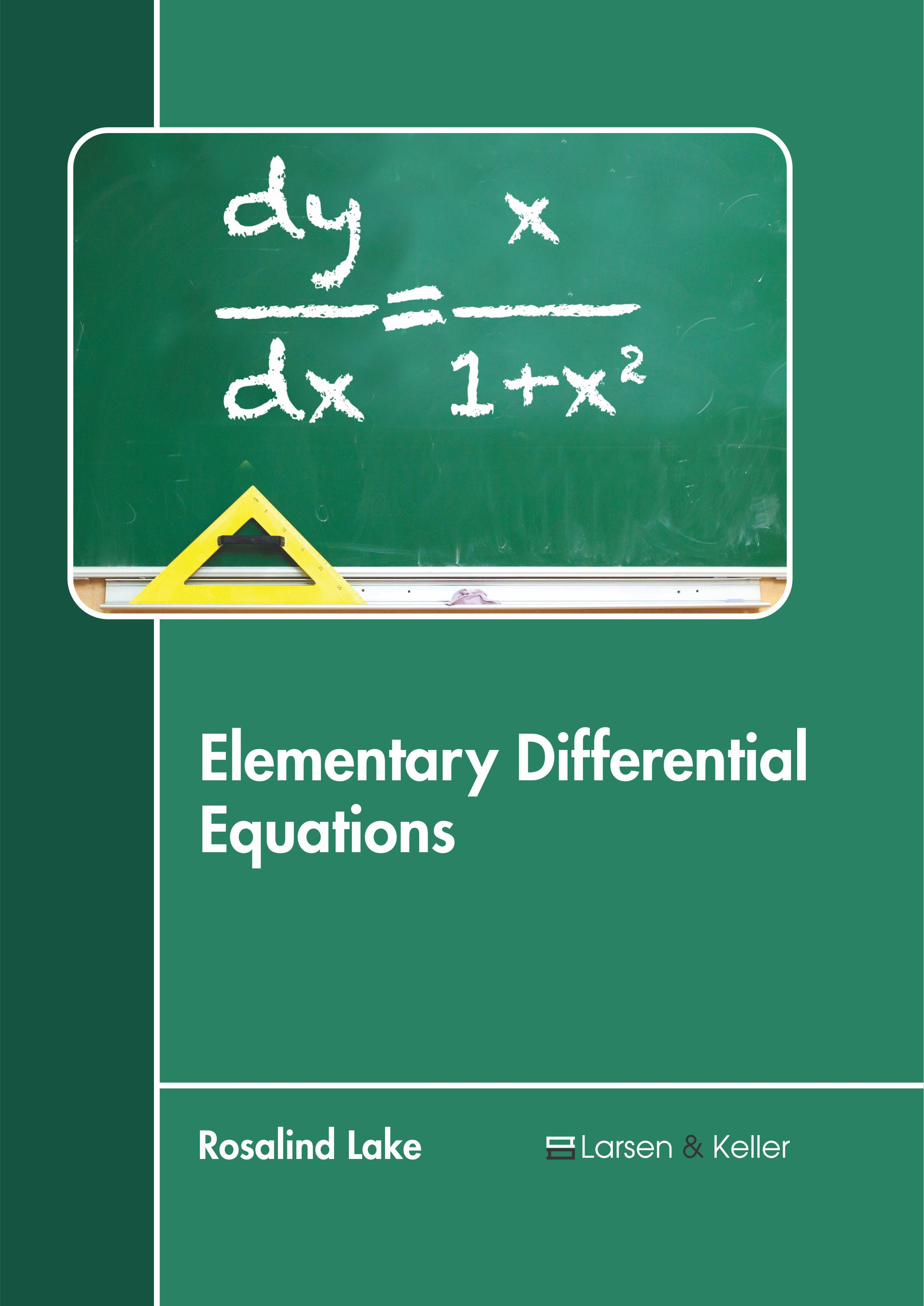BROWSE BY

#### Author

Results 1 - 2 of 2Fundamentals of Differential Equations Author : Rosalind Lake Subject : Mathematics ISBN :9781632386021 The mathematical equations which define the relationship of a function with its derivatives are known as differential equations. The varied types of differential equations include ordinary, partial, non-linear Read MoreElementary Differential Equations Author : Rosalind Lake Subject : Mathematics and Statistics ISBN :9781641721219 A differential equation is a mathematical equation containing functions and their derivatives. A function represents a physical quantity, its derivative expresses the rate of change and the equation describes Read More
Results 1 - 2 of 2